# 音频均衡器 EQfloer rivor

## 概述

EQ（Equaliser）为音频均衡器，通过对声音某一个或多个频段进行增益或者衰减，其他频段保持不变从而达到调整音色的目的。音频均衡器在各个音乐 app 中，各个 RTC 厂商中都可以设置。以声网的 RTC 的 demo 为例，图中红色的部分就是音频均衡器。：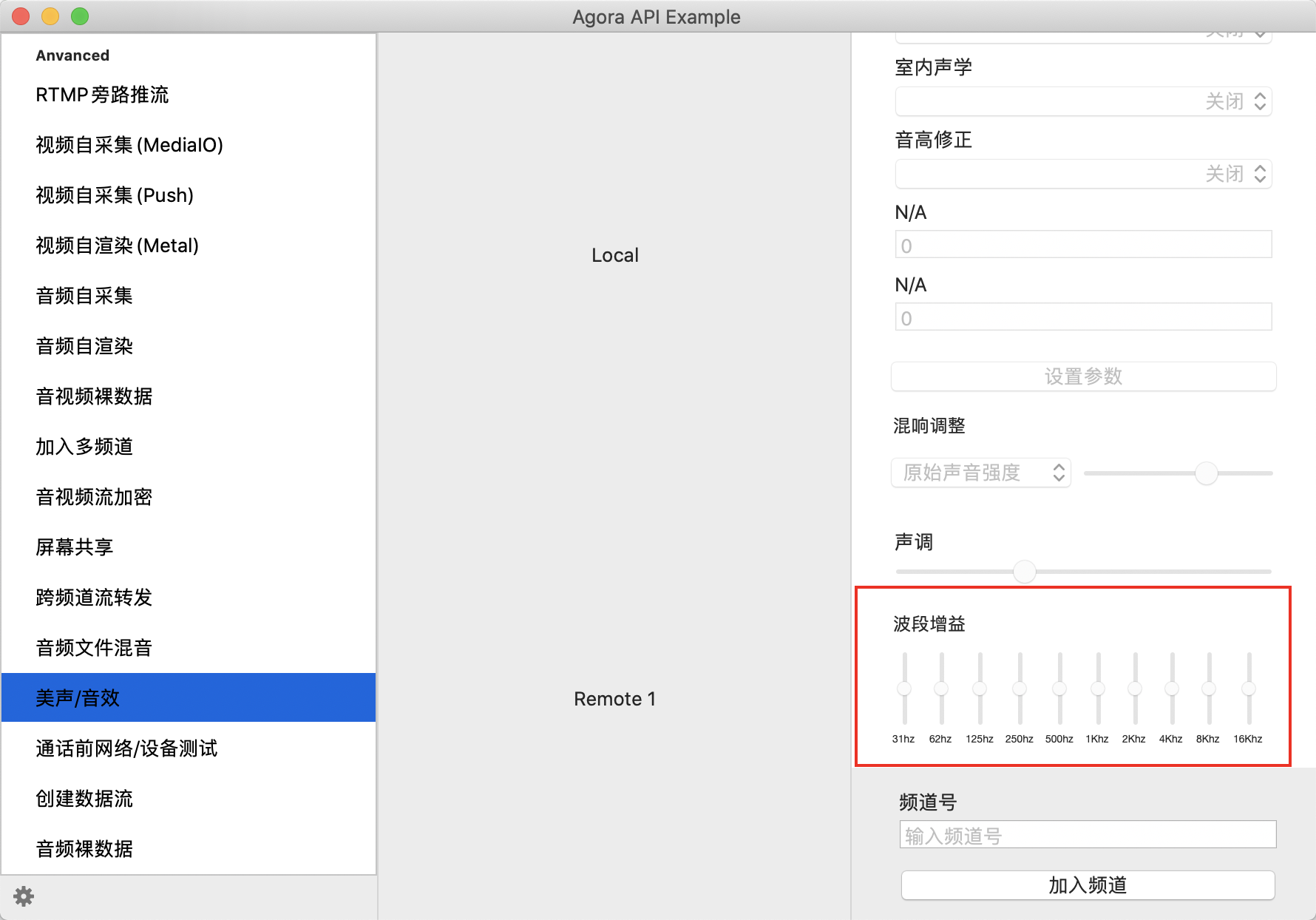### 人声对各个频段的感受

•  HF（高频）：6kHz-16kHz，影响音色的表现力、解析力。

•  MID HF（中高频）：600Hz-6kHz，影响音色的明亮度、清晰度。

•  MID LF（中低频）：200Hz-600Hz，影响音色和力茺和结实度。

•  LF（低频）：20Hz-200Hz，影响音色的混厚度和丰满度。

1. [20－60Hz]影响音色的空间感

2. [60-100Hz]影响声音的混厚感，是低音的基音区。

3. [80－160Hz]主要表现音乐的厚实感，音响在这部分重放效果好的话，会感到音乐厚实、有底气。

4. [150－300Hz]影响声音的力度，尤其是男音的力度。这段频率是男声声音的低频基音频率，同时也是乐音中和弦的根音频率。

5. [300－500Hz]表现人声的（唱歌、朗诵），这个频段上可以表现人声的厚度和力度，好则人声明亮、清晰，否则单薄、混浊。

6. [800Hz]影响音色的力度。如果这个频率丰满，音色会显得强劲有力;如果不足，音色将会显得松弛

7. [1kHz]是音响器材测试的标准参考频率，通常在音响器材中给出的参数是在 1kHz 下测试。这是人耳最为敏感的频率。

8. [1.2kHz]可以适当多一点，不宜太多，可以提高声音的明亮度，过多会使声音发硬。

9. [2k-4kHz]穿透力很强。人耳耳腔的谐振频率是 1∽4KHz，所以人耳对这个频率也是非常敏感的。2-4kHz 对声音的亮度影响很大，这段声音一般不宜衰减。

10. [4k－8kHz]这段频率最影响语音的清晰度、明亮度、如果这频率成分缺少，音色则变得平平淡淡;如果这段频率成分过多，音色则变得尖锐，人声可能出现齿音。

11. [8k-12kHz]这段是音乐的高音区，对音响的高频表现感觉最为敏感。适当突出（一点即可）对音响的的层次和色彩有较大帮助，也会让人感到高音丰富。但是，太多的话会增加背景噪声，同时也会让人感到声音发尖、发毛。如果这段缺乏的话，声音将缺乏感染力和活力。

12. [12k－16kHz]能够影响整体的色彩感，这段过于黯淡会导致乐器失去个性，过多则会产生毛刺感。

13. [16k－20kHz]可能很多人都听不到，听不到并不意味着器材无法回放，只有很少人可以听到 20kHz。这段频率可以影响高频的亮度，以及整体的空间感，过少会让人觉得有点闷，太多则会产生飘忽感，容易产生听觉疲劳。电子合声、古筝钢琴等乐器的泛音多表现于此。

### EQ 参数设置

EQ 的设计原理不复杂，但是如何调节各个波段的增益保持声音动听这是一大难点。EQ 通常使用滤波器来对各个频段滤波来实现。通常我们会把 EQ 设置为 10 个频段，每个频段有中间频率，截止频率，起始频率。在书籍《Audio Effects》有如下表，其中左边代表倍频，右边代表 1/3 倍频：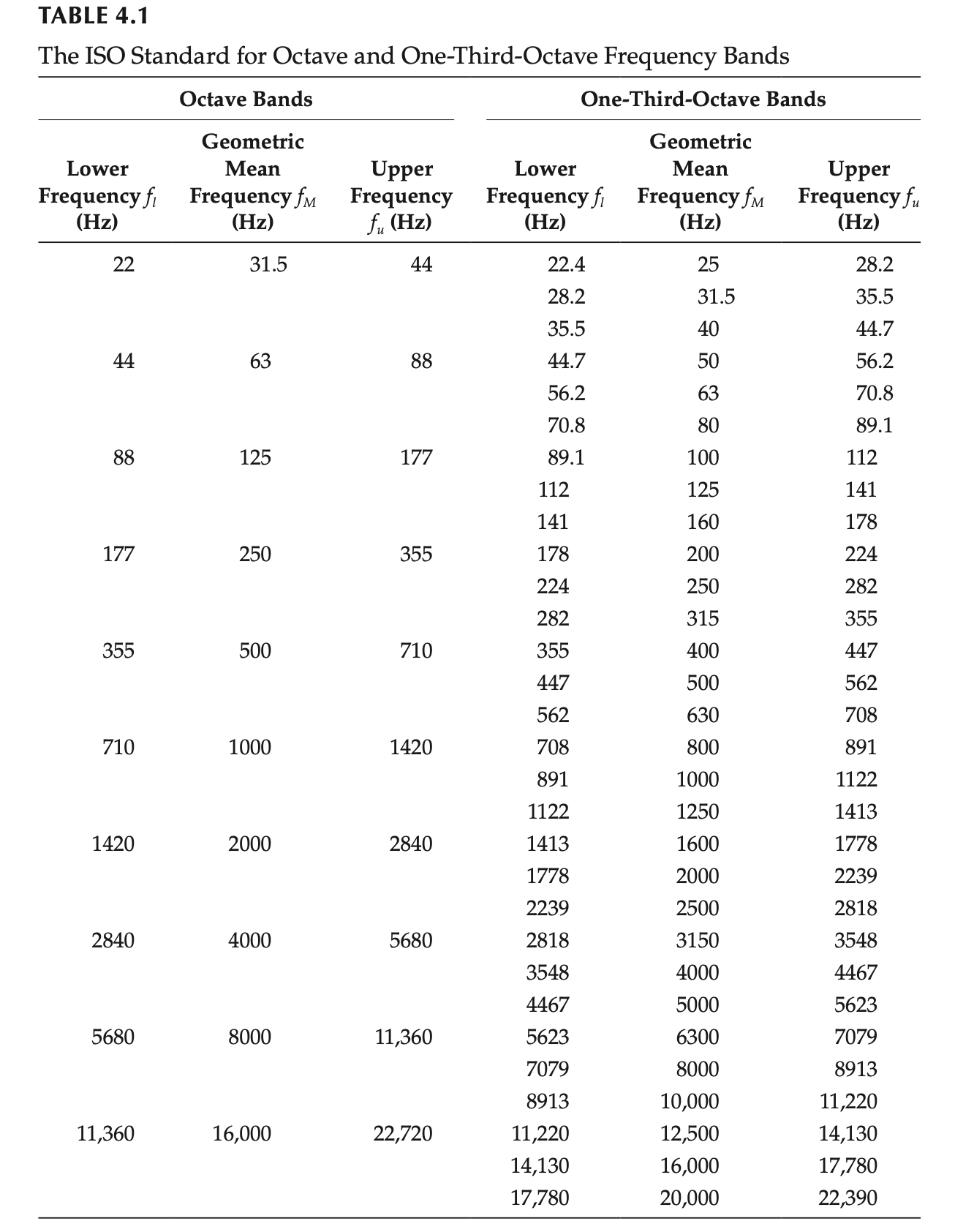1）进行增益的中心频率：f0

2) 当前频段要进行的增益：G Gain 增益可以为正为负

3）设定进行增益或衰减频段宽度的参数：Q Quantize，Q 越小，频段越宽；反之，频段越窄。

## 滤波器

### lowself 滤波器

lowself 滤波器是低通滤波器的一个变形版本，需设置截止频率外增益为 1。因此，lowshelf 滤波器可以认为是低通滤波器在截止频率外并联一个直通信号。

lowself 滤波器的增益曲线为：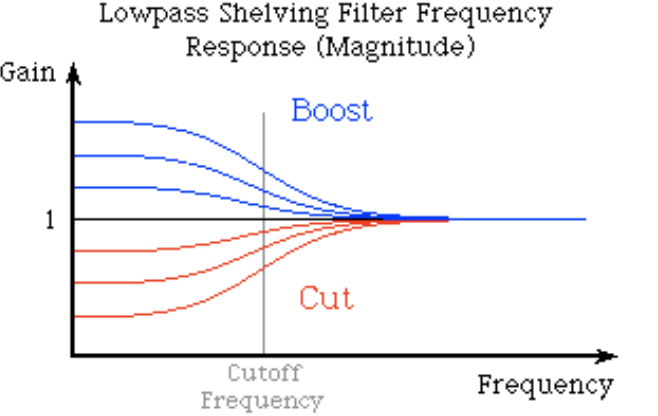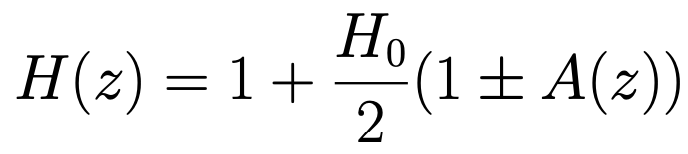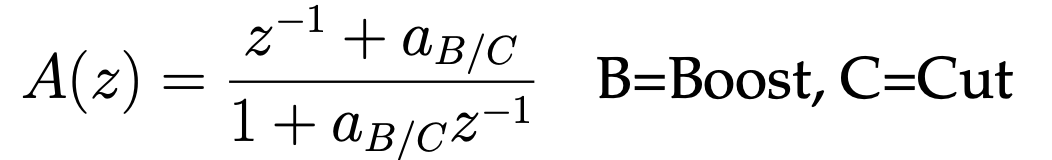### highself 滤波器

highself 滤波器与 lowself 的设计是一样的。只需要将 Z 变化为 1/Z 即可。

highself 滤波器的增益曲线为：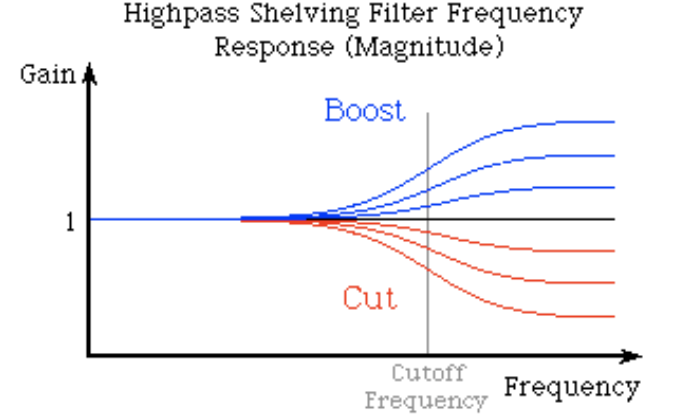### peak 滤波器

peak 滤波器是带通滤波器的变形。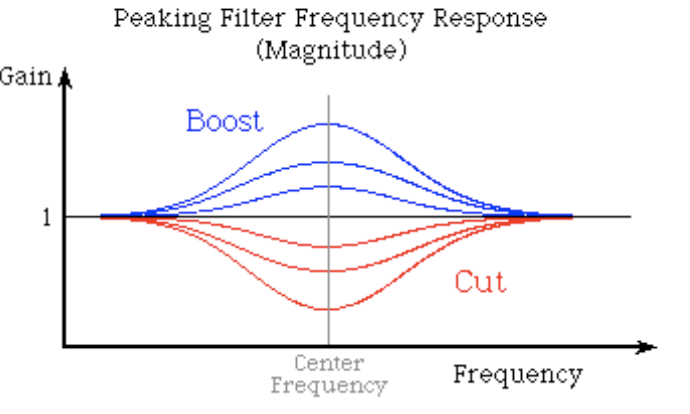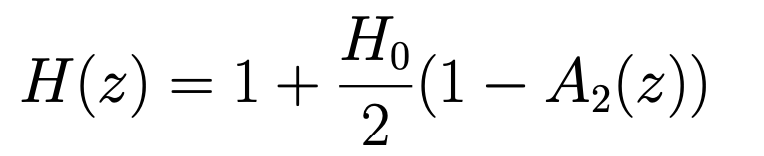## 滤波器实现

b0 + b1*z^-1 + b2*z^-2

H(z) = ------------------------

a0 + a1*z^-1 + a2*z^-2

y[n] = (b0/a0)*x[n] + (b1/a0)*x[n-1] + (b2/a0)*x[n-2]- (a1/a0)*y[n-1] - (a2/a0)*y[n-2]

A = sqrt( 10^(dBgain/20) )=  10^(dBgain/40)w0 = 2*pi*f0/Fsalpha = sin(w0)/(2*Q)                                (case: Q)	  = sin(w0)*sinh( ln(2)/2 * BW * w0/sin(w0) )    (case: BW)	  = sin(w0)/2 * sqrt( (A + 1/A)*(1/S - 1) + 2 )  (case: S）

loweshelf filter 设计为

H(s) = A * (s^2 + (sqrt(A)/Q)*s + A)/(A*s^2 + (sqrt(A)/Q)*s + 1)//计算可得IIR滤波器参数为：                                                     b0 = A*( (A+1) - (A-1)*cos(w0) + 2*sqrt(A)*alpha )b1 = 2*A*( (A-1) - (A+1)*cos(w0) )b2 = A*( (A+1) - (A-1)*cos(w0) - 2*sqrt(A)*alpha )a0 = (A+1) + (A-1)*cos(w0) + 2*sqrt(A)*alphaa1 = -2*( (A-1) + (A+1)*cos(w0) )a2 = (A+1) + (A-1)*cos(w0) - 2*sqrt(A)*alpha

highshelf filter 设计为

H(s) = A * (A*s^2 + (sqrt(A)/Q)*s + 1)/(s^2 + (sqrt(A)/Q)*s + A)    b0 = A*( (A+1) + (A-1)*cos(w0) + 2*sqrt(A)*alpha )b1 = -2*A*( (A-1) + (A+1)*cos(w0) )b2 = A*( (A+1) + (A-1)*cos(w0) - 2*sqrt(A)*alpha )a0 = (A+1) - (A-1)*cos(w0) + 2*sqrt(A)*alphaa1 = 2*( (A-1) - (A+1)*cos(w0) )a2 = (A+1) - (A-1)*cos(w0) - 2*sqrt(A)*alpha

peak filter 设计为

H(s) = (s^2 + s*(A/Q) + 1) / (s^2 + s/(A*Q) + 1)    b0 = 1 + alpha*Ab1 = -2*cos(w0)b2 = 1 - alpha*Aa0 = 1 + alpha/Aa1 = -2*cos(w0)a2 = 1 - alpha/A

## 参考## 评论